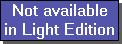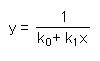The SDL Component Suite is an industry leading collection of components supporting scientific and engineering computing. Please visit the SDL Web site for more information....## CalcReciLinFitUnit: SDL_math2 Class: Declaration: procedure CalcReciLinFit (var k0, k1, FitQual: double);

The procedure CalcReciLinFit calculates the best fitting reciprocal line curve for a given set of data. The curve is determined by the equationThe values of x and y are given by the data samples, the parameters k0, and k1 are estimated by CalcReciLinFit using a least squares approximation. The data points [x,y] have to be entered using the routine EnterStatValue. A minimum number of 3 values is required in order to apply CalcReciLinFit. Do not forget to reset the statistics calculation before entering any new data sets (use the method Init).

In addition to the parameters k0 and k1 CalcReciLinFit returns the goodness of fit FitQual. This parameter may vary between 0.0 and 1.0, indicating the best possible fit if FitQual = 1.0.

 Hint 1: The quality of fit calculated by CalcReciLinFit is not adjusted for the degree of freedoms in the regression parameters.

 Hint 2: The y-values must not be zero. The curve fitting algorithm raises an exception in the case of zero values.

 Example: CURVEFIT.DPR This application lets you draw points in a chart and calculate various types of regression curves. Note, that CURVEFIT.DPR uses the components RCHART and NUMLAB for the user interface, which are not included in the MATH2 package.

 Example: This method is used in the following example program (see http://www.lohninger.com/examples.html for downloading the code): curvefit

Last Update: 2012-Oct-20